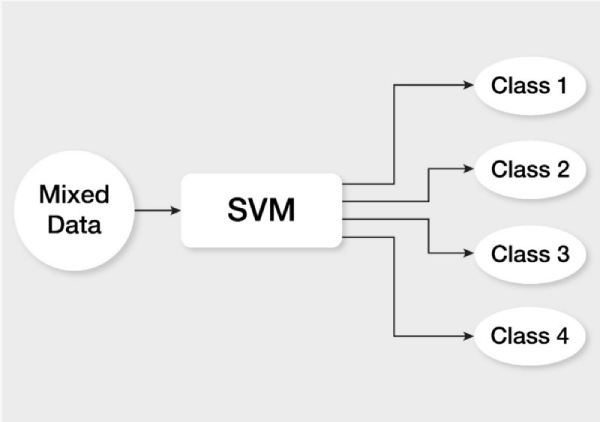• +91-9872993883
• +91-8283824812
• info@ris-ai.com

# Support Vector Machines in Python ¶

## What is Support Vector Machine (SVM)?¶

A Support Vector Machine (SVM) is a supervised Machine Learning Model that uses classification algorithms for two-group classification problems. After giving an SVM model sets of labeled training data for each category, they’re able to categorize new text.

#### >> a fast and dependable classification algorithm that performs very well with a limited amount of data to analyze ¶

At first approximation what SVMs do is to find a separating line(or hyperplane) between data of two classes. SVM is an algorithm that takes the data as an input and outputs a line that separates those classes if possible.SVM tries to make a decision boundary in such a way that the separation between the two classes(that street) is as wide as possible.### STEP WISE representation on how Support Vector Machine (SVM) works in very easy language in given below: ¶

#### 1. Importing Different Libraries ¶

In :
import pandas as pd
from sklearn import svm
from sklearn.model_selection import train_test_split
from sklearn import metrics


#### 2. Read data from csv file ¶

*(Comma Separated Values file) is a type of plain text file that uses specific structuring to arrange tabular data Here is the link to download csv file ( )

In :
dataset = pd.read_csv('Social_Network_Ads.csv')


#### 3. After reading csv file,do partitioning of data of input features and target data ¶

In :
x = dataset.iloc[:, [2, 3]].values  # take features data and
y = dataset.iloc[:, 4].values  # target data


#### 4. We take training dataset as x_train and y_train, and testing data sets as x_test and y_test by taking size 0.3 ¶

In :
x_train, x_test, y_train, y_test = train_test_split(x, y, test_size=0.3)


#### 5. Fit training data in the Support Vector machine present with different Hyperparameters and to predict the output¶ ¶

In :
model = svm.SVC(kernel='linear', C=100, gamma=1)
model.fit(x_train, y_train)
model.score(x_train, y_train)
y_pred = model.predict(x_test)
print(y_pred)

[0 0 0 0 0 1 0 0 0 0 1 0 1 0 0 0 0 0 0 0 0 1 0 0 0 1 1 0 1 0 0 1 0 0 0 1 1
0 0 0 0 0 0 1 0 0 0 0 0 0 0 0 0 0 0 1 0 0 1 0 0 0 1 1 1 1 1 0 0 0 0 0 0 1
0 0 0 0 0 0 0 0 1 0 0 0 1 0 0 0 0 1 1 1 0 0 0 1 0 1 0 0 0 0 0 1 0 0 0 1 1
0 0 0 0 0 0 0 1 0]


### Hyperparameters used in SVM: ¶

#### kernel:¶

kernel parameters selects the type of hyperplane used to separate the data. Using ‘linear’ will use a linear hyperplane (a line in the case of 2D data). ‘rbf’ and ‘poly’ uses a non linear hyper-plane for linear data it always be ‘linear’ and for non linear data it always be ‘rbf’ or ‘poly’.

##### C:¶

It controls the trade off between smooth decision boundary and classifying training points correctly. A large value of c means you will get more training points correctly.

##### Gamma:¶

It defines how far the influence of a single training example reaches. If it has a low value it means that every point has a far reach and conversely high value of gamma means that every point has close reach.

##### 6. For obtaining Accuracy of model code given below is used: ¶
In [ ]:
print("accuracy:", metrics.accuracy_score(y_test, y_pred))


### Conclusion¶

1. A support vector machine allows you to classify data that’s linearly separable.

2. If it isn’t linearly separable, you can use the kernel trick to make it work.

However, for text classification it’s better to just stick to a linear kernel.

###### Compared to newer algorithms like neural networks, they have two main advantages: higher speed and better performance with a limited number of samples (in the thousands). ¶

This makes the algorithm very suitable for text classification problems, where it’s common to have access to a dataset of at most a couple of thousands of tagged samples.